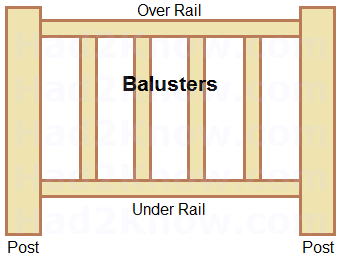# How to Calculate Materials for a Deck Rail

Deck Railing Calculator
Total Length
of Railing
feet
Number of Posts
Post width inches
Baluster Height feet
Baluster Width inches
Spacing Between
Balusters
inches
Number of Balusters:
Length of Board: feet
Number of Screws:
(Amounts include a 4% waste factor.)

Adding a rail to your deck increases its aesthetic appeal and helps create more privacy. If your deck is raised off of the ground, a sturdy railing system helps keep children safe when playing on the deck. Most deck rails are constructed from thick posts with thinner baluster boards in between. If you know the total length of the railing, the number of posts, and the dimensions of the baluster segments, you can estimate the number of screws and wood needed to build a rail.

You can apply the method below to calculate materials, or use the deck railing calculator on the left. If you want to estimate the amount of wood needed to build the frame and top of the deck, use the Deck Materials Calculator

The first step is to find the values for six variables: length of the railing R (feet), number of posts P, width of the posts W (inches), width of the balusters B (inches), spacing between balusters S (inches), and the height of the balusters H (feet).

Once you have measured these values, you can find the number of balusters with this equation:

(12R - PW)/(B + S).

Next, consider the total length of board needed to construct the railing--assuming that you use the same kind of wood for the balusters, over rail, and under rail. The equation is

H(12R - PW)/(B + S) + 2R.

Lastly, the number of screws you need is about

4(12R - PW)/(B + S) + 8(P - 1);

This assumes you use two screws per joint. To be on the safe side, factor in 4% extra for the wood and screws.